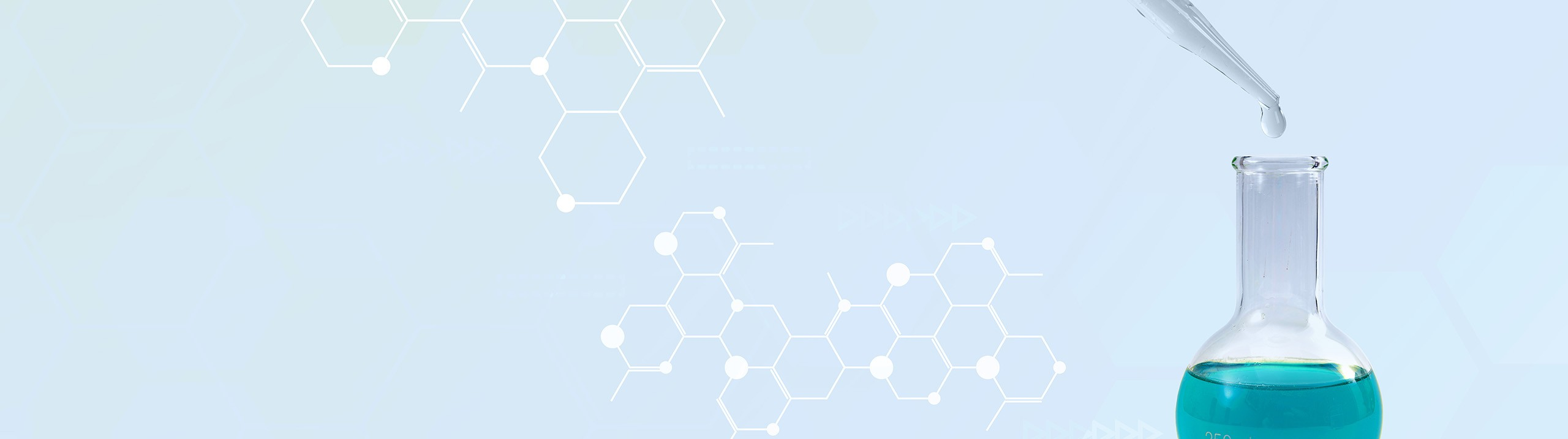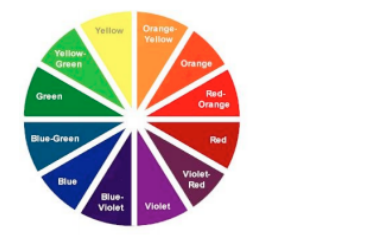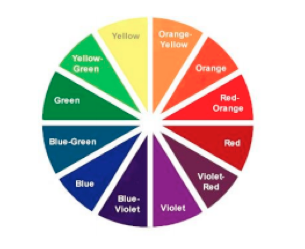Start typing, then use the up and down arrows to select an option from the list.# Analytical Chemistry

Learn the toughest concepts covered in your Analytical Chemistry class with step-by-step video tutorials and practice problems.

Fundamentals of Spectrophotometry

# Measuring Absorbance

## Measuring Absorbance

1

#### example

Measuring Absorbance Calculations 12m
Play a video:
So here it says in UV vis spectroscopy, the absorbent represents the quantity of light that a sample absorbs while the percent trans mittens represents the amount of light that passes through a sample calculate the percent transmits of a substance if its absorbency is measured as 0.419. But we need to realize here is that absorb ints and percent transmit mints are basically opposites of enough of one another. If you are absorbing 100% of light by the substance that means there is 0% of trans mittens that's going on. And then conversely if you are transmitting 100% that means that 0% of light is being absorbed. Okay so they kind of work against each other here we're going to say that percent transmitting. So um percent t equals your anti log 2 to the -, absorb its. Okay so that's the formula we use in order to determine what our transmits will be. So then what we're gonna say here is we're gonna say 10 To the to -119. So that's going to equal here 10 to the 1.581. So then when we punch that in we're gonna get here 38.1. So that represents 38.1%. So that means that we're going to have 38.1% of light passing through the sample which means that the remainder of 100% is being absorbed by that sample. So you'd say absorb ints would be just 100% minus that number. Remember they're opposites of one another. If one is doing its job 100% The other one would be 0% and you gotta find a way of balancing the two. In terms of your sample itself, the more concentrated your sample becomes, the harder it is for light to pass through it, so the more it'll absorb light Since we've done that one. Move on to the next question. Here we say. If your percent, transmissions is 39.1% determine the absorbent. Pretty straightforward. We're going the opposite way. See if you can manipulate this equation to get the desired equation to figure out your absorbent.
2

#### example

Measuring Absorbance Calculations 11m
Play a video: